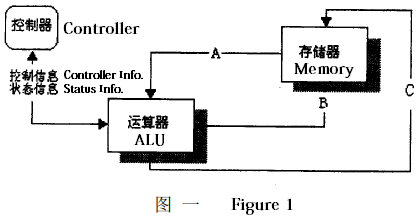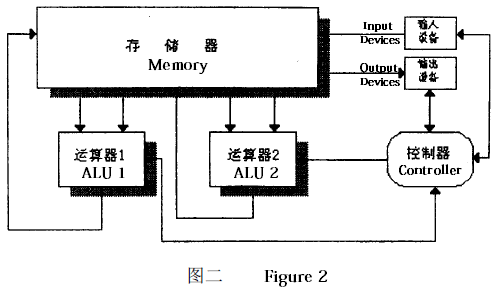## Day 2, Problem 3 - Parallel Computing

The arithmetic logic unit (ALU) is an important component in computers due to its function of performing mathematical calculations. Figure 1 is a simplified depiction of how an ALU operates. To perform one calculation, the controller instructs the ALU to accept two source operands A and B from the memory unit. After a certain amount of time, C, the resulting value of the computation, is sent back and written to a specified location in the memory unit.The time it takes the ALU to perform a certain operation is given by the following table.

Operation TypeOperationOperation Time
2C = A − BTsub
3C = A × BTmul
4C = A ÷ BTdiv

When evaluating complex mixed operation expressions, the ALU turns into a "bottleneck" of high-speed computing. To achieve faster computation results, computer scientists have designed a computer with two ALUs running in parallel. Its structure is depicted in figure 2.The ability for the two ALUs to perform calculations at the same time drastically improves the speed of the entire computer. The parallel computer has two types of commands:

The operation command: `OP Time Alu_no Operate_no Address1 Address2 Address3`

• The keyword `OP` is followed by six parameters:
• `Time` indicates the time that the command was issued.
• `Alu_no` is the ID of the ALU that should bear the operation (`Alu_no` ∈ {1, 2}).
• `Operate_no` is the type of operation being performed (`Operate_no` ∈ {1, 2, 3, 4}).
• `Address1`, `Address2` are the addresses of the two operands in the memory unit.
• `Address3` is the address in the memory unit at which the result of the operation should be stored.

The termination command: `END Time Address`

• The keyword `END` is followed by two parameters:
• `Time` is the time when the entire computation process should terminate.
• `Address` is the address in memory at which the final computation result should be stored.

Each ALU can only process a single operation command at a time, and the termination command ends all computation.

You are to write a program for the controller that, given an expression to evaluate, directs the parallel computer depicted in figure 2 to compute the correct value for the expression while minimizing the total computation time.

### Input Format

The first line of input consists of four positive integers, each no larger than 1000, the values of Tadd, Tsub, Tmul, and Tdiv. The second line of input contains the mixed-operation expression (no longer than 255 characters). Each variable in the expression will consist of a single uppercase alphabetical letter. The initial values for the variables will be stored in consecutive memory addresses 1, 2, … according to alphabetical order (e.g. if the expression only uses the variables A, B, and D, then address 1 will initially store the value of A, 2 will store B, and 3 will store D).

### Output Format

The output should contain the optimal commands for evaluating the expression, in order of when they are issued (commands issued at the same time can be given in any order), one command per line.

### Guarantees

The initial time when the controller process may start is 0.
Time values in this problem will all be in the same unit.
There are at most 1000 available addresses in the memory unit (at locations 1 through 1000).
The time for reading from and writing to the memory unit is negligible.
If both ALUs simultaneously try to operate on the memory unit, then ALU 1 always operates before ALU 2.

### Sample Input

```2 2 4 12
C+(A+B)*C-E/F+F
```

### Sample Output

```OP 0 1 1 1 2 6
OP 0 2 4 4 5 8
OP 2 1 1 3 5 7
OP 4 1 3 6 3 10
OP 8 1 1 10 7 11
OP 12 1 2 11 8 12
END 14 12
```

Point Value: 25 (partial)
Time Limit: 1.00s
Memory Limit: 16M